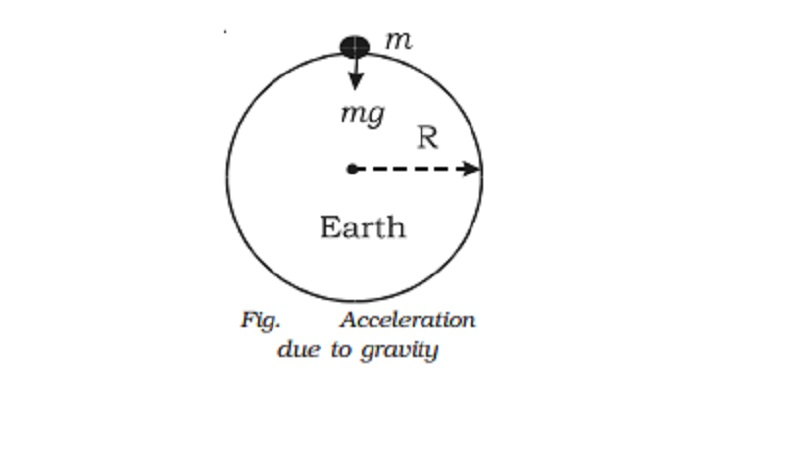Home | | Physics | | Physics | Acceleration due to gravity at the surface of the Earth

# Acceleration due to gravity at the surface of the EarthConsider a body of mass m on the surface of the Earth as shown in the Fig.. Its distance from the centre of the Earth is R (radius of the Earth). The gravitational force experienced by the body is F = GMm / R2 where M is the mass of the Earth.

Acceleration due to gravity at the surface of the Earth

Consider a body of mass m on the surface of the Earth as shown in the Fig.. Its distance from the centre of the Earth is R (radius of the Earth).

The gravitational force experienced by the body is F = GMm / R2 where M is the mass of the Earth.

From Newton's second law of motion,

Force F = mg.

Equating the above two forces, GMm/R2 = mg

G=GM/R2

This equation shows that g is independent of the mass of the body m. But, it varies with the distance from the centre of the Earth. If the Earth is assumed to be a sphere of radius R, the value of g on the surface of the Earth is given by g = GM/R2.

Mass of the Earth

From the expression g = GM/R2 mass of the Earth can be calculated as follows :

M =gR2/G =  5.98 � 1024 kg

Acceleration due to gravity

Galileo was the first to make a systematic study of the motion of a body under the gravity of the Earth. He dropped various objects from the leaning tower of Pisa and made analysis of their motion under gravity. He came to the conclusion that 'in the absence of air, all bodies will fall at the same rate'. It is the air resistance that slows down a piece of paper or a parachute falling under gravity. If a heavy stone and a parachute are dropped where there is no air, both will fall together at the same rate.

Experiments showed that the velocity of a freely falling body under gravity increases at a constant rate. (i.e) with a constant acceleration. The acceleration produced in a body on account of the force of gravity is called acceleration due to gravity. It is denoted by g. At a given place, the value of g is the same for all bodies irrespective of their masses. It differs from place to place on the surface of the Earth. It also varies with altitude and depth.

The value of g at sealevel and at a latitude of 45o is taken as the standard (i.e) g = 9.8 m s-2

Study Material, Lecturing Notes, Assignment, Reference, Wiki description explanation, brief detail

Related Topics